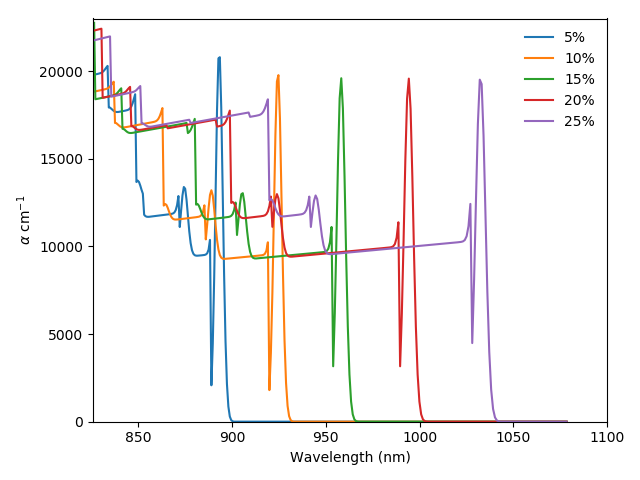# Absorption of quantum wells¶

For modelling the optical properties of QWs we use the method described by S. Chuang (). The absorption coefficient at thermal equilibrium in a QW is given by: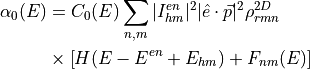where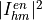is the overlap integral between the holes in level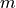and the electrons in level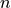;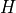is a step function,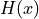= 1 for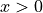, 0 and 0 for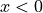,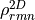is the 2D joint density of states,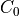a proportionality constant dependent on the energy, and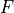the excitonic contribution, which will be discussed later.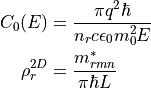Here,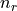is the refractive index of the material,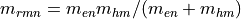the reduced, in-plane, effective mass and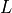an effective period of the quantum wells. The in-plane effective mass of each type of carriers is calculated for each level, accounting for the spread of the wavefunction into the barriers as ():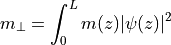This in-plane effective mass is also used to calculate the local density of states shown in Figure [fig:qw]b. In Eq. [eq:QW_abs2],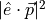is the momentum matrix element, which depends on the polarization of the light and on the Kane’s energy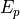, specific to each material and determined experimentally. For band edge absorption, where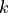= 0, the matrix elements for the absorption of TE and TM polarized light for the transitions involving the conduction band and the heavy and light holes bands are given in Table [tab:matrix_elements]. As can be deduced from this table, transitions involving heavy holes cannot absorb TM polarised light.

TE

TM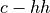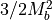0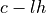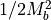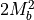Table: Momentum matrix elements for transitions in QWs.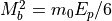is the bulk matrix element.

In addition to the band-to-band transitions, QWs usually have strong excitonic absorption, included in Eq. [eq:qw_abs] in the term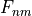. This term is a Lorenzian (or Gaussian) defined by an energy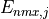and oscillator strength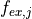. It is zero except for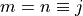where it is given by Klipstein et al. ():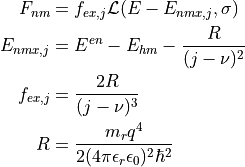Here,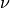is a constant with a value between 0 and 0.5 and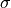is the width of the Lorentzian, both often adjusted to fit some experimental data. In Solcore, they have default values of= 0.15 and= 6 meV.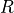is the exciton Rydberg energy ().

Fig. [fig:QW_absorption] shows the absorption coefficient of a range of InGaAs/GaAsP QWs with a GaAs interlayer and different In content. Higher indium content increases the depth of the well, allowing the absorption of less energetic light and more transitions.